# Multiplying 3 digit by 1 digit number

Multiplying 3 digit by 1 digit number – Question 1

Multiply :
132 x 2 = ?

Explanation

Step I: Multiply 2 in ones place by 2
2 x 2 = 4
Write 4 in ones place.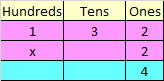Step II: Multiply 3 in tens place by 2
3 x 2 = 6
Write 6 in tens place.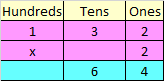Step III: Multiply 1 in hundreds place by 2
1 x 2 = 2
Write 2 in hundreds place.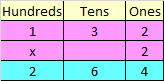Hence, 132 x 2 = 264

Multiplying 3 digit by 1 digit number – Question 2

Multiply :
465 x 2 = ?

Explanation

Step I: Multiply 5 in ones place by 2
5 x 2 = 10
Since, the number is more than 9, we need to carry over 1 to the tens place
Write 0 in ones place.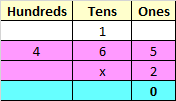Step II: Multiply 6 in tens place by 2
6 x 2 = 12
Add the carry over of 1 to 12
12 + 1 = 13
Since, the number is more than 9, we need to carry over 1 to the hundreds place
Write 3 in tens place.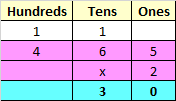Step III: Multiply 4 in hundreds place by 2
4 x 2 = 8
Add the carry over of 1 to 8
8 + 1 = 9
Write 9 in hundreds place.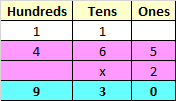Hence, 465 x 2 = 930